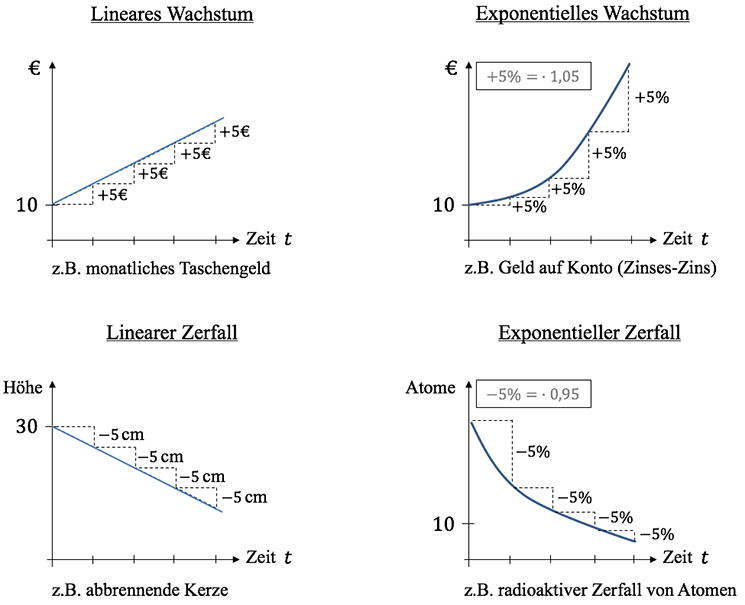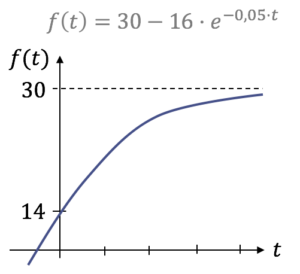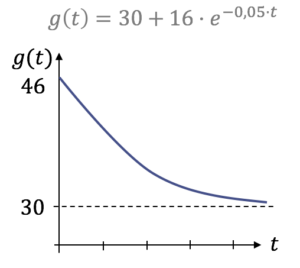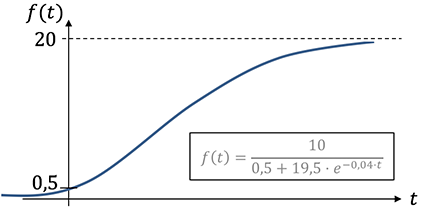# The growth is always faster than exponential

## Growth processes

Calculations for growth or growth processes deal with the development of a stock. An important idea here is that the change in the stock (i.e. increase and decrease) is the derivation of the stock.

Table of Contents

Our Mathe-Abi'21 learning booklets Explanations ✔ Examples ✔ Free learning videos ✔

New!

### Linear growth processes

The linear growth is very, very simple. This is a stock with a steady development! So the same amount always comes in (or goes away) in every period of time. Linear growth processes are described by straight lines, so the approach is:

\ begin {align *}
y = m \ cdot x + b \ quad \ textrm {or} \ quad B (t) = m \ cdot t + b
\ end {align *}

### example

In a pond, which initially contains 200 m \$ ^ 3 \$ dirty, smelly water, 4 m \$ ^ 3 \$ clean, crystal clear water flows into it every day.

1. How much water does the lake contain after 50 days?

Linear growth is simply described by our well-known straight line equation. Since growth processes mostly depend on the time \$ t \$ (English for "time"), you often also \$ B (t) = m \ cdot t + b \$. Here the stock \$ B \$ depends on the time \$ t \$. \$ b \$ denotes the stock at time 0, \$ m \$ the increase per time unit \$ t \$. So we can describe our example as follows:

\ begin {align *}
B (t) = 4 \ cdot t + 200 \ quad [\ textrm {m} ^ 3]
\ end {align *}

To find out how much water there is after 50 days, we plug \$ t = 50 \$ into the above equation and get:

\ begin {align *}
B (50) = 4 \ cdot 50+ 200 = 400 \ \ textrm {m} ^ 3
\ end {align *}

Answer: After 50 days there are 400 m \$ ^ 3 \$ in the pool.

2. When does the lake contain 1000 m \$ ^ 3 \$ of water?

Solution 1 - Consider: At the beginning there were already 200 m \$ ^ 3 \$ in the pool, so \$ 1000-200 = 800 \$ m \$ ^ 3 \$ were added. Since 4 m \$ ^ 3 \$ are added daily, I need 800/4 = 200 days so that 1000 m \$ ^ 3 \$ are in the pool.

Solution 2 - use equation: The stock \$ B \$ should be 1000 m \$ ^ 3 \$. So we put 1000 in the straight line equation and convert according to the unknown \$ t \$. It follows:

\ begin {align *}
B (t) & = 4 \ cdot t + 200 \
1000 & = 4 \ cdot t + 200 \ quad \ Rightarrow \ quad t = 200 \ quad [\ textrm {days}]
\ end {align *}

3. When is only 1% of the water dirty?

At this point we think about it and look at the text of the exercise. Only clean water flows into it. The only dirty water in the pool is the initial stock. So the searched \$ 1 \% \$ are the initial \$ 200 \$ m \$ ^ 3 \$. With the help of the rule of three we can find out that \$ 100 \% \$ must be \$ 20000 \$ m \$ ^ 3 \$.

Now the question arises, when is \$ 20,000 m \$ ^ 3 \$ in the pond. We can solve this in the same way as part 2. We use the second approach here and get:

\ begin {align *}
B (t) & = 4 \ cdot t + 200 \
20000 & = 4 \ cdot t + 200 \ quad \ Rightarrow \ quad t = 4950 \ quad [\ textrm {days}]
\ end {align *}

Take a closer look at the learning video on the subject of linear growth processes.

Linear growth alone as a basis, then exponential growth, Math by Daniel Jung

Math Abi'21 study booklet incl. Exercise collection

New!

### Exponential growth processes

In the previous chapter we learned what linear growth is all about. We have deliberately omitted the representation of the linear decay because the processes are identical. The only difference is that something always decreases instead of increasing by the same amount.

Exponential growth processes are processes in which the increase (or decrease) is always proportional to the stock, i.e. the same percentage is always added to the existing stock (or goes away). Standard example: Interest at the bank (the same interest rate is always added to an invested capital).

Exponential growth processes are given by the functional equation

\ begin {align *}
\ textrm {end value} \ & = \ \ textrm {start value} \ cdot \ textrm {base} ^ x \
f (x) & = s \ cdot b ^ x \
f (t) & = a \ cdot q ^ {t}
\ end {align *}

With

\ begin {align *}
& q> 1 \ \ textrm {as a growth factor} \
\ textrm {and} \ & q <1 \ \ textrm {as decay factor}
\ end {align *}

described.

What does that mean now? Here some examples:

• 200 flies double their number every day: \$ f (t) = 200 \ cdot 2 ^ {t} \$
• 200 flies halve their number every day: \$ f (t) = 200 \ cdot 0.5 ^ {t} \$
• 200 flies reproduce daily by \$ 7 \% \$. General: \$ f (t) = a \ cdot \ left (1+ \ frac {p} {100} \ right) ^ {t} \$

\ begin {align *}
f (t) = 200 \ cdot \ left (1+ \ frac {7} {100} \ right) ^ t = 200 \ cdot 1.07 ^ t
\ end {align *}

• 200 flies are reduced by \$ 5% a day. General: \$ f (t) = a \ cdot \ left (1- \ frac {p} {100} \ right) ^ {t} \$

\ begin {align *}
f (t) = 200 \ cdot \ left (1- \ frac {5} {100} \ right) ^ t = 200 \ cdot 0.95 ^ t
\ end {align *}

The following illustration should serve as an overview of the topic of growth processes. Here, linear and exponential processes are compared so that the differences can be clearly seen.

Our Mathe-Abi'21 learning booklets Explanations ✔ Examples ✔ Free learning videos ✔

New!This is typical for exponential growth processes Doubling time or. Generation time, where it asks when the double starting value (or starting balance) will be reached and the Half-life (with exponential decrease), where the question is asked when half the starting value (or starting balance) will be reached. Since double the start value (\$ 2 \ cdot S \$) is always asked for with \$ S \$ as the start value for the doubling time, there is always a 2 or 0.5 for the half-life on the left-hand side of the equation.

### Examples:

Generation time:

\ begin {align *}
f (t) & = 200 \ cdot 1.05 ^ t \
400 & = 200 \ cdot 1.05 ^ t \ quad |: 200 \
2 & = 1.05 ^ t \ quad | \ textrm {with log} \
t & = \ log_ {1.05} (2)
\ end {align *}

Half-life:

\ begin {align *}
f (t) & = 200 \ cdot 0.8 ^ t \
100 & = 200 \ cdot 0.8 ^ t \ quad |: 200 \
0.5 & = 0.8 ^ t \ quad | \ textrm {with log} \
t & = \ log_ {0.8} \ left (0.5 \ right)
\ end {align *}

### e-function and growth processes

If the basis of the exponential function is Euler's number \$ e \$, then we speak of THE exponential function. The base \$ e \$ is often chosen for tasks on growth or decay processes. The general form is:

\ begin {align *}
f (t) & = a \ cdot e ^ {\ pm k \ cdot t} \
\ textrm {with} \ quad \ quad k & = ln (1+ \ frac {p} {100}) \ quad \ textrm {as growth constant and} \
k & = ln (1- \ frac {p} {100}) \ quad \ textrm {as a decay constant.}
\ end {align *}

Set up an exponential function with 2 points

Often the tasks in growth processes are set in such a way that two points have to be found out of the task text and an exponential function has to be set up from these two points. Let's take a look at a typical example.

### Example:

Daniel has a normal hormone level of 6 mg / l. When he saw Chantal for the first time, the hormone level jumped to 9 mg / l within 3 minutes. How high is the hormone level after a quarter of an hour if one can assume a development according to \$ h (t) = a \ cdot e ^ {kt} \$?

What are we going to do? The form of the function, here exponential function, is already given. The following information must be extracted from the task:

\ begin {align *}
t & = 0: \ h (0) = 6 \ quad \ textrm {from this follows the point} \ P_1 (0 | 6) \
t & = 3: \ h (3) = 9 \ quad \ textrm {from this follows the point} \ P_2 (3 | 9) \
\ end {align *}

Find the hormone level after a quarter of an hour, i.e. \$ h (15) =? \$.
Two equations then follow from \$ P_1 (0 | 6) \$ and \$ P_2 (3 | 9) \$, which we solve for the two unknowns \$ a \$ and \$ k \$ using the methods known to us.

\ begin {align *}
\ textrm {I} \ quad 6 & = a \ cdot \ underbrace {e ^ {k \ cdot 0}} _ {= 1} \
\ textrm {II} \ quad 9 & = a \ cdot e ^ {k \ cdot 3}
\ end {align *}

From the equation \$ \ textrm {I} \$ it follows directly \$ a = 6 \$ and we insert that into the equation \$ \ textrm {II} \$ and get:

\ begin {align *}
9 & = 6 \ cdot e ^ {k \ cdot 3} \ quad |: 6 \
\ Leftrightarrow \ quad 1,5 & = e ^ {3k} \ quad | \ \ ln \
\ Rightarrow \ quad \ ln (1,5) & = 3k \ quad |: 3 \
\ Leftrightarrow \ quad k & = \ frac {\ ln (1.5)} {3} \ approx 0.135
\ end {align *}

This means that the growth function we are looking for is: \$ h (t) = 6 \ cdot e ^ {0.135 \ cdot t} \$. If you have the function, the rest is mostly easy. Daniel has a hormone level of after 15 minutes

\ begin {align *}
h (15) = 6 \ cdot e ^ {0.135 \ cdot 15} \ approx 45.46 \ \ frac {\ textrm {mg}} {\ textrm {l}}.
\ end {align *}

Please note that the rounding errors in \$ e \$ functions are very high.

Daniel explains everything about exponential growth processes to you again in his learning video.

Exponential growth, overviews, also decay | Math by Daniel Jung, explanatory video

Math Abi'21 study booklet incl. Exercise collection

New!

### Unlimited growth processes or unlimited disintegration

In this section you should get an overview of unlimited growth / decay. We have \$ N (t) \$ as the \$ y \$ value that comes out when I have an initial value
\$ N (0) \$ times \$ e ^ {k \ cdot t} \$. \$ k \$ must be greater than zero. It does not matter whether there is growth or decay.

Unlimited growth:

\ begin {align *}
N (t) & = N (0) \ cdot e ^ {k \ cdot t} \
N '(t) & = k \ cdot N (0) \ cdot e ^ {k \ cdot t} \
& = k \ cdot N (t)
\ end {align *}

Doubling time: \$ t = \ frac {\ ln (2)} {k} \$

Unlimited decay:

\ begin {align *}
N (t) & = N (0) \ cdot e ^ {- k \ cdot t} \
N '(t) & = - k \ cdot N (0) \ cdot e ^ {- k \ cdot t} \
& = - k \ cdot N (t)
\ end {align *}

Half-life: \$ t = \ frac {\ ln (0.5)} {- k} \$

### Half-life example

In living organisms, the proportion of the carbon isotope C14 is about one trillionth of all carbon atoms. The C14 isotope decays exponentially in dead organisms. After 1000 years there are still around 0.886 trillionths left. Determine the half-life of C14.

There is unlimited decay. Our approach is initially \$ N (t) = N (0) \ cdot e ^ {- kt} \$. So we have to determine the initial value \$ N (0) \$ and \$ k \$.
2 Information is given in the exercise text to determine the values ​​you are looking for:

• Initial value: \$ N (0) = 1 \$
• After 1000 years: \$ N (1000) = \$ 0.886

Note: The unit is a trillionth. We obtain

\ begin {align *}
0.886 & = 1 \ cdot e ^ {- k \ cdot 1000} \ quad | \ ln \
\ ln (0.886) & = - k \ cdot 1000 \
k & = 0.000121
\ end {align *}

and thus the function that describes the unlimited decay: \$ N (t) = 1 \ cdot e ^ {- 0.000121 \ cdot t} \$.
Only now do we begin with the question. We just use the formula from above and it follows for the half-life:

\ begin {align *}
t = \ frac {\ ln (0.5)} {- 0.000121} \ approx 5728 \ \ textrm {years}
\ end {align *}

Check out the tutorial on exponential, unlimited growth processes!

Exponential, unlimited growth, decay | Math by Daniel Jung

Math Abi'21 study booklet incl. Exercise collection

New!

### Limited growth processes and limited acceptance

A basic distinction is made between limited growth and limited decrease. In general, with \$ k> 0 \$, \$ s \ in \ mathbb {R} \$ and \$ t \$ for the time:

\ begin {align *}
f (t) = S \ pm c \ cdot e ^ {- k \ cdot t}
\ end {align *}

Limited growth process:Example of restricted growth: You take a glass of milk out of the refrigerator and put it in your room. We have an increase in temperature that is limited to room temperature.

Limited purchaseExample of limited purchase: You heat a glass of milk and put it in your room. We have a decrease in temperature that is limited to room temperature.
It is characteristic of limited growth or limited decrease that the slope decreases with increasing time. Difference to logistical growth!

For more information, watch Daniel's video on Restricted Growth Processes.

Limited growth, limited decrease | Math by Daniel Jung

Math Abi'21 study booklet incl. Exercise collection

New!

### Logistic growth processes

Similar to limited growth, if you look to the right, you will see that the slope of the graph continues to decrease until it is 0 and asymptotically approaches a limit value. In contrast to limited growth, however, the speed of growth increases at the beginning before it decreases. In the illustration you can see an example of how a logistical growth could look graphically.How do you get to the limit value (limit \$ S \$), which is 20 here? To put it simply: number above by number that stands on its own is the so-called limit at which the graph levels off in terms of value. In our example: \$ 10 / 0.5 = \$ 20.
The number that stands alone under the fraction line is also the point of intersection with the \$ y \$ axis. Here: \$ 0.5. \$ This has clarified all the peculiarities.
In general, the following equation applies to logistical growth:

\ begin {align *}
f (t) = \ frac {a \ cdot S} {a + (S-a) \ cdot e ^ {- S kt}},
\ end {align *}

where \$ a = f (0) \$, \$ 0 0 \$ and \$ S> 0 \$.

Math Abi'21 study booklet incl. Exercise collection

New!

### Larger growth / decay period

If a longer period than \ textit {daily, hourly, minute} is given, e.g. every 4 days 200g will be 20 \% more, you have two options for setting up the exponential function:

Possibility Number 1:
Calculate the correct growth factor!

\ begin {align *}
f (x) & = 200 \ cdot q ^ x \
\ underbrace {240} _ {200 + 20 \%} & = 200 \ cdot q ^ 4 \ quad |: 200 \
1,2 & = q ^ 4 \ quad \ quad \ quad \ | \ textrm {4. Take root} \
q & = \ sqrt  {1,2} \
\ Rightarrow \ f (x) & = 200 \ cdot \ left (\ sqrt  {1,2} \ right) ^ x
\ end {align *}

Option 2:

Adjust exponents!

\ begin {align *}
f (x) & = 200 \ cdot (\ underbrace {1,2} _ {1+ \ frac {0,2} {100}}) ^ {\ frac {1} {4} x} \
& = 200 \ cdot \ left (1,2 ^ {\ frac {1} {4}} \ right) ^ x \
& = 200 \ cdot \ left (\ sqrt  {1,2} \ right) ^ x
\ end {align *}

Our Mathe-Abi'21 learning booklets Explanations ✔ Examples ✔ Free learning videos ✔

New!